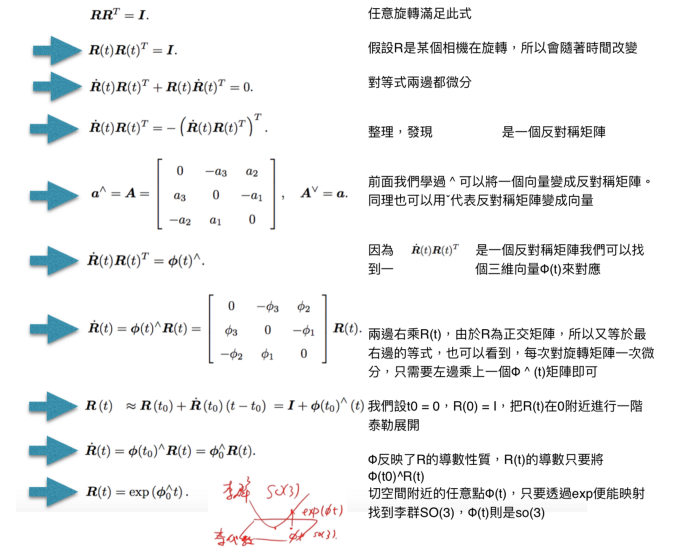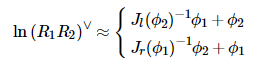# 1. 旋转点求导

$f'(x) = \lim_{\Delta x\rightarrow0}\frac{f(x+\Delta x)}{\Delta x}$

• 用李代数（旋转向量）来表示姿态，然后利用李代数加法叠加微小量并对该微小量进行求导

• 李代数求导：在李群对应的李代数的局部坐标上，即：（ $\boldsymbol{\phi}, \boldsymbol{\phi}^\wedge\in \mathfrak{so}(3)$ ） 上添加扰动，即： $\boldsymbol{\phi} \leftarrow \boldsymbol{\phi} + \delta\boldsymbol{\phi}$ ，由于李代数本身对应旋转向量，因此对旋转向量添加扰动相当于同时改变旋转轴和旋转角度。
• 用李群（旋转矩阵）表示姿态，然后左/右乘上一个扰动，然后对该扰动求导，即左扰动模型和右扰动模型

• 旋转矩阵右扰动求导：由于旋转矩阵没有加法，因此要对旋转矩阵本身添加扰动，需要先通过指数映射将李代数转化为李群，然后根据李群的运算来添加扰动，即： $\boldsymbol{R} \leftarrow \boldsymbol{R}\exp{(\boldsymbol{\phi}^\wedge)}$ ，由于是旋转矩阵右乘扰动，因此相当于是在局部坐标系下对旋转矩阵进行更新
• 旋转矩阵左扰动求导：和右扰动同理，我们也可以将扰动添加在旋转矩阵左侧，即： $\boldsymbol{R} \leftarrow \exp{(\boldsymbol{\phi}^\wedge)}\boldsymbol{R}$ ，由于是旋转矩阵左乘扰动，因此相当于在全局坐标系下对旋转矩阵进行更新

# 2 $\boldsymbol{J}_{\boldsymbol{R}_0}$是什么

$\boldsymbol{e}(\boldsymbol{R}, \boldsymbol{p}) = \boldsymbol{e}(\boldsymbol{R}_0, \boldsymbol{p}) + \boldsymbol{J}_{\boldsymbol{R}_0}\delta\boldsymbol{\phi}$

# 3 $\oplus$、$\wedge$ 和 $_{\times}$ 的含义

$\hat{\xi}$ $\xi$ 的对应李代数，当然也可以像上面表示为 $\xi^{\wedge}$

$\exp{\xi}$ 为李代数到李群的指数映射；

$\hat{\xi} = [\omega t]_{\times}$ 为李代数，李代数可以转化为反对称矩阵。通过 $_{\times}$ 来表示角度扰动（旋转轴+旋转角度）的旋转向量。# 5 连乘李群的求导

• 右扰动模型

• 左扰动模型

• 右扰动模型

• 左扰动模型# 6. 参考链接

https://hermit.blog.csdn.net/article/details/121034589

https://zhuanlan.zhihu.com/p/356409543

https://xiaotaoguo.com/p/gtsam-math-derivatives/